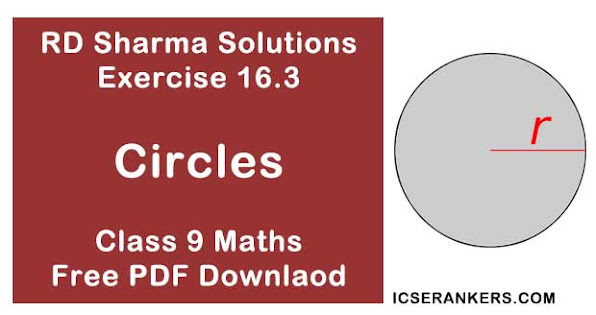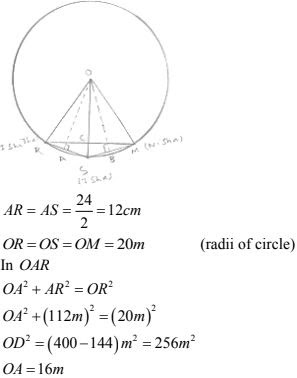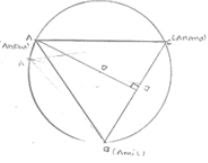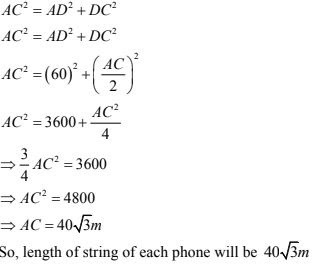# Chapter 16 Circles RD Sharma Solutions Exercise 16.3 Class 9 MathsChapter Name RD Sharma Chapter 16 Circles Exercise 16.3 Book Name RD Sharma Mathematics for Class 10 Other Exercises Exercise 16.1Exercise 16.2Exercise 16.4Exercise 16.5 Related Study NCERT Solutions for Class 10 Maths

### Exercise 16.3 Solutions

1. Three girls Ishita, Isha and Nisha are playing a game by standing on a circle of radius 20 m drawn in a park. Ishita throws a ball o Isha, Isha to Nisha and Nisha to Ishita. If the distance between Ishita and Isha and between Isha and Nisha is 24 m each, what is the distance between Ishita and Nisha.

Solution

Let R, S and M be the position of Ishita, Isha and Nasha respectivelyWKT, in an isosceles triangle altitude divides the base, So in ΔRSM , ∠RCS will be 90° and
RC = CM.

Area of ΔORS = 1/2 × OA × RS
⇒ 1/2 × RC × OS = 1/2 × 16 × 24
⇒ RC × 20 = 16 × 24 ⇒ RC = 192 ⇒ RM = 2(192) = 38.4 m
So, distance between Ishita and Nisha is 384 m.

2. A circular park of radius 40 m is situated in a colony. Three boys Ankur, Amit and Anand are sitting at equal distance on its boundary each having a toy telephone in his hands to talk to each other. Find the length of the string of each phone.

SolutionGiven that AB = BC = CA
So, ABC is an equilateral triangle